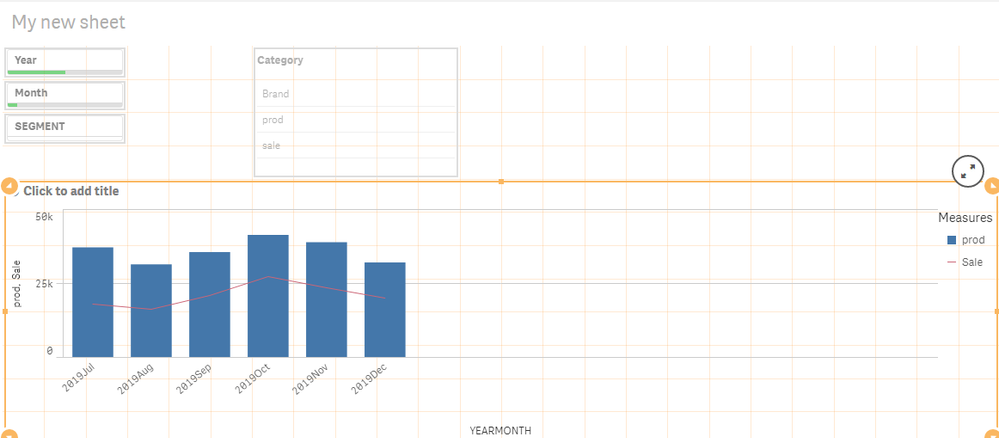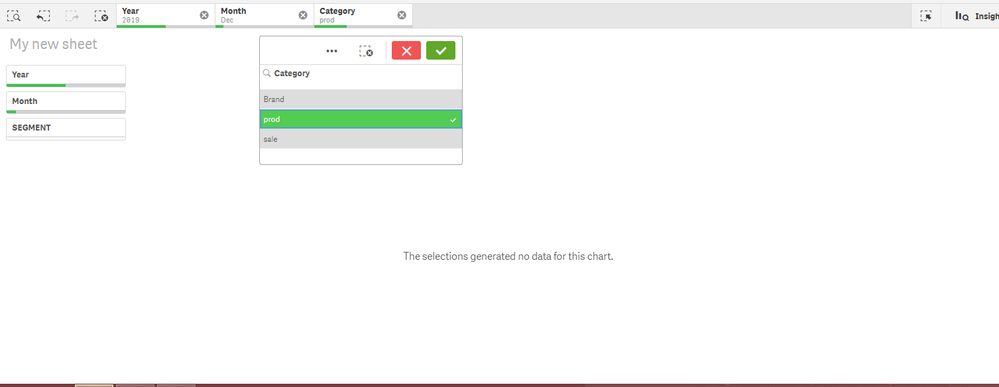# QlikView App Dev

Discussion Board for collaboration related to QlikView App Development.

Announcements
Join this live chat April 6, 10AM EST - QlikView to Qlik Sense REGISTER
cancel
Showing results for
Search instead for
Did you mean:Specialist

## How to write condition enabling in expression

Hello All,

i Need help in doing a condition enabling in a chart , in below combo chart by default i have to show as below

Dimension: YEARMONTH

Expression: 1)Prod:    Sum({<Month,Year,Date={">=\$(=date(monthstart(addmonths(max(Date),-5)),'DD-MM-YYYY'))<=\$(=date(((max(Date))),'DD-MM-YYYY'))"}>}p_input)

2)Sale:      Sum({<Month,Year,Date={">=\$(=date(monthstart(addmonths(max(Date),-5)),'DD-MM-YYYY'))<=\$(=date(((max(Date))),'DD-MM-YYYY'))"}>}p_d_op)

Combo:so far so good,

Now my requirement is to do a condition enabling , I have created a inline field called Category which as values prod,sale,Brand and in each expression i have added condition as shown below

Combo Chart:

Dimension: YEARMONTH

EXpression :1)   if(Getselectedcount(Category) =0 or Category='prod',

Sum({<Month,Year,Date={">=\$(=date(monthstart(addmonths(max(Date),-5)),'DD-MM-YYYY'))<=\$(=date(((max(Date))),'DD-MM-YYYY'))"}>}p_input)

)

Before selecting CategoryAfter selecting  prod from categoryI am getting only Selected Year, Month prod values even after ignoring Year,Month

Kindly suggest,how to write condition in expression.

Thanks

Labels (2)

• ### sunny_talwar

1 Solution

Accepted SolutionsMVP

New expressions

prod

``````If(SubStringCount(Concat(DISTINCT TOTAL {1<Category = p(Category)>} '|' & Category & '|'), '|prod|'),
Sum({<Month, Year, Date = {">=\$(=Date(MonthStart(Max(Date),-5), 'DD-MM-YYYY'))<=\$(=Date(Max(Date), 'DD-MM-YYYY'))"}>} p_input)
)``````

sale

``````If(SubStringCount(Concat(DISTINCT TOTAL {1<Category = p(Category)>} '|' & Category & '|'), '|sale|'),
Sum({<Month, Year, Date = {">=\$(=Date(MonthStart(Max(Date), -5), 'DD-MM-YYYY'))<=\$(=Date(Max(Date), 'DD-MM-YYYY'))"}>} p_d_OP)
)``````
28 RepliesEmployee

What are you expecting to see?

You are filtering on Category='prod', the chart is showing the MonthYear when 'prod' is soldSpecialist
Author

Hello,

Yes ur right i am filtering on Category='prod ' and the chart is showing only prod bar,

the issue is, it is showing only 2019Dec bar where as it suppose to show last six months bars i mean

from 2019Dec to 2019 jul,

Even though the current selections are Year =2019 and Month=Dec, But i have bypass those selections in my expression to show last six months bars.Specialist
Author

Any suggestion on how to write condition in expression plzSpecialist
Author

Hello All , Kindly suggest on the above requirement plzContributor

Designer has built-in operators that can be used in enabling conditions or validations UPSers.Specialist
Author

HI, what exactly u mean to say, what is designer?Specialist
Author

Still ,i couldn't find any solution for the above requirement . Please share your toughs  on the requirement ..MVP

Try this

``````If(GetSelectedCount(Category) = 0 or Only({1} Category) = 'prod',

Sum({<Month,Year,Date={">=\$(=Date(MonthStart(Max(Date), -5), 'DD-MM-YYYY'))<=\$(=Date(Max(Date), 'DD-MM-YYYY'))"}>} p_input)

)``````Specialist
Author

Hi sunny ,

Thanks for the reply ....i have written expressions as below

1)Expression : prod

If(GetSelectedCount(Category) = 0 or Only({1} Category) = 'prod',

Sum({<Month,Year,Date={">=\$(=Date(MonthStart(Max(Date), -5), 'DD-MM-YYYY'))<=\$(=Date(Max(Date), 'DD-MM-YYYY'))"}>} p_input)

)

2)Expression : sale

If(GetSelectedCount(Category) = 0 or Only({1} Category) = 'sale',

Sum({<Month,Year,Date={">=\$(=date(monthstart(addmonths(max(Date),-5)),'DD-MM-YYYY'))<=\$(=date(((max(Date))),'DD-MM-YYYY'))"}>}p_d_OP)
)

Before selecting any value from category :After selecting  prod from category :I am getting select either prod or sale or both.......